Time normalization of data¶

Marcos Duarte
Laboratory of Biomechanics and Motor Control (http://demotu.org/)
Federal University of ABC, Brazil

Time normalization is usually employed for the temporal alignment of cyclic data obtained from different trials with different duration (number of points). The most simple and common procedure for time normalization used in Biomechanics and Motor Control is known as the normalization to percent cycle (although it might not be the most adequate procedure in certain cases (Helwig et al., 2011).

In the percent cycle, a fixed number (typically a temporal base from 0 to 100%) of new equally spaced data is created based on the old data with a mathematical procedure known as interpolation.
Interpolation is the estimation of new data points within the range of known data points. This is different from extrapolation, the estimation of data points outside the range of known data points.
Time normalization of data using interpolation is a simple procedure and it doesn't matter if the original data have more or less data points than desired.

The Python function tnorm.py (code at the end of this text) implements the normalization to percent cycle procedure for time normalization. The function signature is:

yn, tn, indie = tnorm(y, axis=0, step=1, k=3, smooth=0, mask=None,
nan_at_ext='delete', show=False, ax=None)

Let's see now how to perform interpolation and time normalization; first let's import the necessary Python libraries and configure the environment:

In :
# Import the necessary libraries
import numpy as np
import matplotlib.pyplot as plt
%matplotlib inline
import sys
sys.path.insert(1, r'./../functions')  # add to pythonpath

For instance, consider the data shown next. The time normalization of these data to represent a cycle from 0 to 100%, with a step of 1% (101 data points) is:

In :
y = [5,  4, 10,  8,  1, 10,  2,  7,  1,  3]
print("y data:")
y
y data:
Out:
[5, 4, 10, 8, 1, 10, 2, 7, 1, 3]
In :
t  = np.linspace(0, 100, len(y))  # time vector for the original data
tn = np.linspace(0, 100, 101)     # new time vector for the new time-normalized data
yn = np.interp(tn, t, y)          # new time-normalized data
print("y data interpolated to 101 points:")
yn
y data interpolated to 101 points:
Out:
array([ 5.  ,  4.91,  4.82,  4.73,  4.64,  4.55,  4.46,  4.37,  4.28,
4.19,  4.1 ,  4.01,  4.48,  5.02,  5.56,  6.1 ,  6.64,  7.18,
7.72,  8.26,  8.8 ,  9.34,  9.88,  9.86,  9.68,  9.5 ,  9.32,
9.14,  8.96,  8.78,  8.6 ,  8.42,  8.24,  8.06,  7.58,  6.95,
6.32,  5.69,  5.06,  4.43,  3.8 ,  3.17,  2.54,  1.91,  1.28,
1.45,  2.26,  3.07,  3.88,  4.69,  5.5 ,  6.31,  7.12,  7.93,
8.74,  9.55,  9.68,  8.96,  8.24,  7.52,  6.8 ,  6.08,  5.36,
4.64,  3.92,  3.2 ,  2.48,  2.15,  2.6 ,  3.05,  3.5 ,  3.95,
4.4 ,  4.85,  5.3 ,  5.75,  6.2 ,  6.65,  6.88,  6.34,  5.8 ,
5.26,  4.72,  4.18,  3.64,  3.1 ,  2.56,  2.02,  1.48,  1.02,
1.2 ,  1.38,  1.56,  1.74,  1.92,  2.1 ,  2.28,  2.46,  2.64,
2.82,  3.  ])

The key is the Numpy interp function, from its help:

interp(x, xp, fp, left=None, right=None)
One-dimensional linear interpolation.
Returns the one-dimensional piecewise linear interpolant to a function with given values at discrete data-points.

A plot of the data will show what we have done:

In :
plt.figure(figsize=(10,5))
plt.plot(t, y, 'bo-', lw=2, label='original data')
plt.plot(tn, yn, '.-', color=[1, 0, 0, .5], lw=2, label='time normalized')
plt.legend(loc='best', framealpha=.5)
plt.xlabel('Cycle [%]')
plt.show()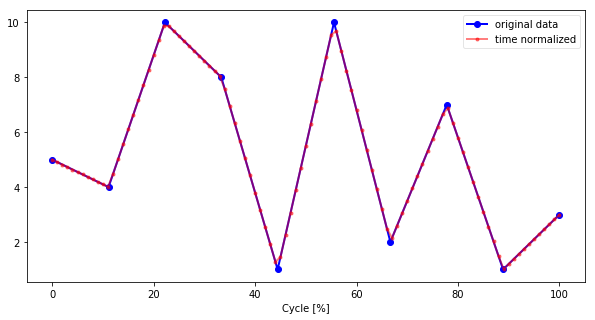The function tnorm.py implements this kind of normalization with option for a different interpolation than the linear one used, deal with missing points in the data (if these missing points are not at the extremities of the data because the interpolation function can not extrapolate data), other things.
Let's see the tnorm.py examples:

In :
from tnorm import tnorm
In :
>>> # Default options: cubic spline interpolation passing through
>>> # each datum, 101 points, and no plot
>>> y = [5,  4, 10,  8,  1, 10,  2,  7,  1,  3]
>>> tnorm(y)
Out:
(array([  5.        ,   4.17809249,   3.5387693 ,   3.06958033,
2.75807549,   2.59180468,   2.55831781,   2.64516477,
2.83989546,   3.13005979,   3.50320766,   3.94688897,
4.44865363,   4.99605153,   5.57663259,   6.17794669,
6.78754374,   7.39297365,   7.98178632,   8.54153165,
9.05975953,   9.52401988,   9.9218626 ,  10.24155044,
10.47700754,  10.62485776,  10.68174158,  10.6442995 ,
10.509172  ,  10.27299957,   9.93242271,   9.4840819 ,
8.92461763,   8.25067039,   7.46097301,   6.57858161,
5.64224479,   4.6909727 ,   3.76377547,   2.89966325,
2.13764617,   1.51673437,   1.075938  ,   0.85426719,
0.89073208,   1.22148967,   1.83147733,   2.66132882,
3.65021709,   4.73731509,   5.86179577,   6.96283209,
7.97959699,   8.85126343,   9.51700436,   9.91599272,
9.98916876,   9.73107126,   9.19820454,   8.45052464,
7.54798763,   6.55054957,   5.5181665 ,   4.51079448,
3.58838957,   2.81090783,   2.2383053 ,   1.92987303,
1.90500085,   2.12123175,   2.53078856,   3.08589411,
3.73877122,   4.44164272,   5.14673143,   5.8062602 ,
6.37245184,   6.79752918,   7.0338503 ,   7.05573627,
6.88356441,   6.54351135,   6.06175374,   5.46446819,
4.77783135,   4.02801986,   3.24121034,   2.44357944,
1.66130378,   0.92056   ,   0.24752473,  -0.33162538,
-0.79071371,  -1.10356362,  -1.24399848,  -1.18584166,
-0.90291651,  -0.3690464 ,   0.4419453 ,   1.55623522,   3.        ]),
array([   0.,    1.,    2.,    3.,    4.,    5.,    6.,    7.,    8.,
9.,   10.,   11.,   12.,   13.,   14.,   15.,   16.,   17.,
18.,   19.,   20.,   21.,   22.,   23.,   24.,   25.,   26.,
27.,   28.,   29.,   30.,   31.,   32.,   33.,   34.,   35.,
36.,   37.,   38.,   39.,   40.,   41.,   42.,   43.,   44.,
45.,   46.,   47.,   48.,   49.,   50.,   51.,   52.,   53.,
54.,   55.,   56.,   57.,   58.,   59.,   60.,   61.,   62.,
63.,   64.,   65.,   66.,   67.,   68.,   69.,   70.,   71.,
72.,   73.,   74.,   75.,   76.,   77.,   78.,   79.,   80.,
81.,   82.,   83.,   84.,   85.,   86.,   87.,   88.,   89.,
90.,   91.,   92.,   93.,   94.,   95.,   96.,   97.,   98.,
99.,  100.]),
[0, 9])
In :
>>> # Linear interpolation passing through each datum
>>> yn, tn, indie = tnorm(y, k=1, smooth=0, mask=None, show=True)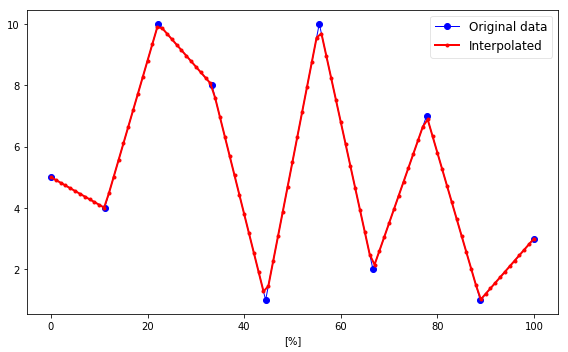In :
>>> # Cubic spline interpolation with smoothing
>>> yn, tn, indie = tnorm(y, k=3, smooth=1, mask=None, show=True)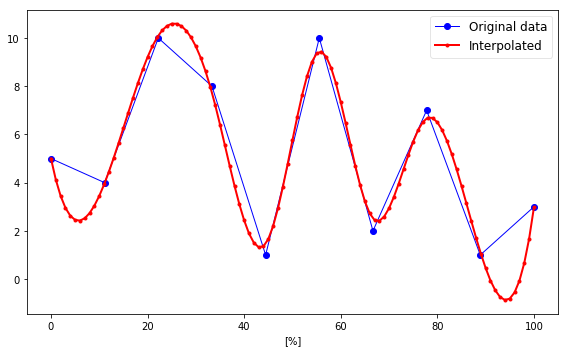In :
>>> # Cubic spline interpolation with smoothing and 50 points
>>> x = np.linspace(-3, 3, 60)
>>> y = np.exp(-x**2) + np.random.randn(60)/10
>>> yn, tn, indie = tnorm(y, step=-50, k=3, smooth=1, show=True)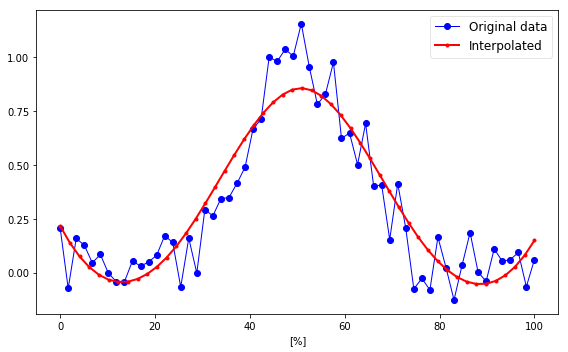In :
>>> # Deal with missing data (use NaN as mask)
>>> x = np.linspace(-3, 3, 100)
>>> y = np.exp(-x**2) + np.random.randn(100)/10
>>> y[:10] = np.NaN # first ten points are missing
>>> y[30: 41] = np.NaN # make other 10 missing points
>>> yn, tn, indie = tnorm(y, step=-50, k=3, smooth=1, show=True)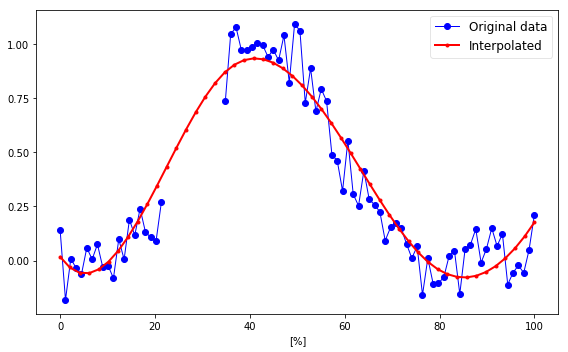In :
>>> # Deal with missing data at the extremities replacing by first/last not-NaN
>>> x = np.linspace(-3, 3, 100)
>>> y = np.exp(-x**2) + np.random.randn(100)/10
>>> y[0:10] = np.NaN # first ten points are missing
>>> y[-10:] = np.NaN # last ten points are missing
>>> yn, tn, indie = tnorm(y, step=-50, k=3, smooth=1, nan_at_ext='replace', show=True)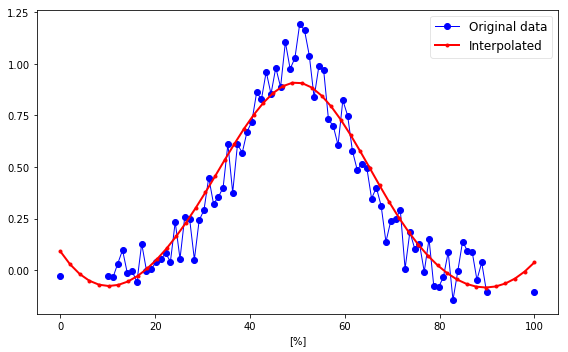In :
>>> # Deal with missing data at the extremities replacing by first/last not-NaN
>>> x = np.linspace(-3, 3, 100)
>>> y = np.exp(-x**2) + np.random.randn(100)/10
>>> y[0:10] = np.NaN # first ten points are missing
>>> y[-10:] = np.NaN # last ten points are missing
>>> yn, tn, indie = tnorm(y, step=-50, k=1, smooth=0, nan_at_ext='replace', show=True)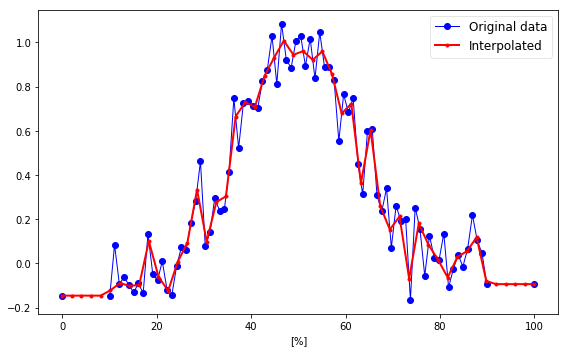In :
>>> # Deal with 2-D array
>>> x = np.linspace(-3, 3, 100)
>>> y = np.exp(-x**2) + np.random.randn(100)/10
>>> y = np.vstack((y-1, y[::-1])).T
>>> yn, tn, indie = tnorm(y, step=-50, k=3, smooth=1, show=True)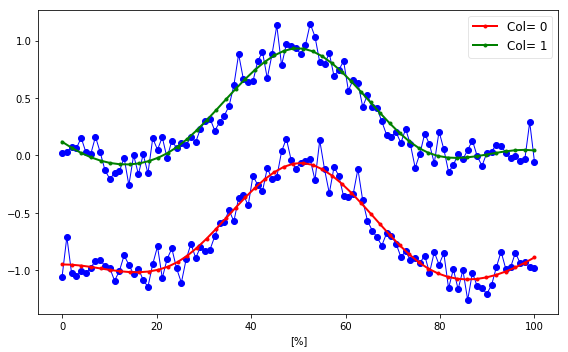Function tnorm.py¶

In [ ]:
"""Time normalization (from 0 to 100% with step interval)."""

import numpy as np

__author__ = 'Marcos Duarte, https://github.com/demotu/BMC'
__version__ = "1.0.6"

def tnorm(y, axis=0, step=1, k=3, smooth=0, mask=None, nan_at_ext='delete',
show=False, ax=None):
"""Time normalization (from 0 to 100% with step interval).

Time normalization is usually employed for the temporal alignment of data
obtained from different trials with different duration (number of points).
This code implements a procedure knwown as the normalization to percent
cycle.

This code can perform simple linear interpolation passing through each
datum or spline interpolation (up to quintic splines) passing through each
datum (knots) or not (in case a smoothing parameter > 0 is inputted).

NaNs and any value inputted as a mask parameter and that appears at the
extremities might be removed or replaced by the first/last not-NaN value
before the interpolation because this code does not perform extrapolation.
For a 2D array, the entire row with NaN or a mask value at the extermity
might be removed because of alignment issues with the data from different
columns. As result, if there is a column of only NaNs in the data, the
time normalization can't be performed (an empty NaNs and any value
inputted as a mask parameter and that appears in the middle of the data
(which may represent missing data) are ignored and the interpolation is
performed through these points.

See this IPython notebook _.

Parameters
----------
y : 1-D or 2-D array_like
Array of independent input data. Must be increasing.
If 2-D array, the data in each axis will be interpolated.
axis : int, 0 or 1, optional (default = 0)
Axis along which the interpolation is performed.
0: data in each column are interpolated; 1: for row interpolation
step : float or int, optional (default = 1)
Interval from 0 to 100% to resample y or the number of points y
should be interpolated. In the later case, the desired number of
points should be expressed with step as a negative integer.
For instance, step = 1 or step = -101 will result in the same
number of points at the interpolation (101 points).
If step == 0, the number of points will be the number of data in y.
k : int, optional (default = 3)
Degree of the smoothing spline. Must be 1 <= k <= 5.
If 3, a cubic spline is used.
The number of data points must be larger than k.
smooth : float or None, optional (default = 0)
Positive smoothing factor used to choose the number of knots.
If 0, spline will interpolate through all data points.
If None, smooth=len(y).
mask : None or float, optional (default = None)
Mask to identify missing values which will be ignored.
It can be a list of values.
NaN values will be ignored and don't need to be in the mask.
nan_at_ext : string, optional (default = 'delete')
Method to deal with NaNs at the extremities.
'delete' will delete any NaN at the extremities (the corresponding
entire row in y for a 2-D array).
'replace' will replace any NaN at the extremities by first/last
not-NaN value in y.
show : bool, optional (default = False)
True (1) plot data in a matplotlib figure.
False (0) to not plot.
ax : a matplotlib.axes.Axes instance, optional (default = None).

Returns
-------
yn : 1-D or 2-D array
Interpolated data (if axis == 0, column oriented for 2-D array).
tn : 1-D array
New x values (from 0 to 100) for the interpolated data.
inds : list
Indexes of first and last rows without NaNs at the extremities of y.
If there is no NaN in the data, this list is [0, y.shape-1].

Notes
-----
This code performs interpolation to create data with the desired number of
points using a one-dimensional smoothing spline fit to a given set of data
points (scipy.interpolate.UnivariateSpline function).

References
----------
..  http://www.sciencedirect.com/science/article/pii/S0021929010005038
..  http://nbviewer.ipython.org/github/demotu/BMC/blob/master/notebooks/TimeNormalization.ipynb

--------
scipy.interpolate.UnivariateSpline:
One-dimensional smoothing spline fit to a given set of data points.

Examples
--------
>>> # Default options: cubic spline interpolation passing through
>>> # each datum, 101 points, and no plot
>>> y = [5,  4, 10,  8,  1, 10,  2,  7,  1,  3]
>>> tnorm(y)

>>> # Linear interpolation passing through each datum
>>> y = [5,  4, 10,  8,  1, 10,  2,  7,  1,  3]
>>> yn, tn, indie = tnorm(y, k=1, smooth=0, mask=None, show=True)

>>> # Cubic spline interpolation with smoothing
>>> y = [5,  4, 10,  8,  1, 10,  2,  7,  1,  3]
>>> yn, tn, indie = tnorm(y, k=3, smooth=1, mask=None, show=True)

>>> # Cubic spline interpolation with smoothing and 50 points
>>> x = np.linspace(-3, 3, 100)
>>> y = np.exp(-x**2) + np.random.randn(100)/10
>>> yn, tn, indie = tnorm(y, step=-50, k=3, smooth=1, show=True)

>>> # Deal with missing data (use NaN as mask)
>>> x = np.linspace(-3, 3, 100)
>>> y = np.exp(-x**2) + np.random.randn(100)/10
>>> y[:10] = np.NaN # first ten points are missing
>>> y[30: 41] = np.NaN # make other 10 missing points
>>> yn, tn, indie = tnorm(y, step=-50, k=3, smooth=1, show=True)

>>> # Deal with missing data at the extremities replacing by first/last not-NaN
>>> x = np.linspace(-3, 3, 100)
>>> y = np.exp(-x**2) + np.random.randn(100)/10
>>> y[0:10] = np.NaN # first ten points are missing
>>> y[-10:] = np.NaN # last ten points are missing
>>> yn, tn, indie = tnorm(y, step=-50, k=3, smooth=1, nan_at_ext='replace', show=True)

>>> # Deal with missing data at the extremities replacing by first/last not-NaN
>>> x = np.linspace(-3, 3, 100)
>>> y = np.exp(-x**2) + np.random.randn(100)/10
>>> y[0:10] = np.NaN # first ten points are missing
>>> y[-10:] = np.NaN # last ten points are missing
>>> yn, tn, indie = tnorm(y, step=-50, k=1, smooth=0, nan_at_ext='replace', show=True)

>>> # Deal with 2-D array
>>> x = np.linspace(-3, 3, 100)
>>> y = np.exp(-x**2) + np.random.randn(100)/10
>>> y = np.vstack((y-1, y[::-1])).T
>>> yn, tn, indie = tnorm(y, step=-50, k=3, smooth=1, show=True)

Version history
---------------
'1.0.6':
Deleted 'from __future__ import ...'
Added parameter nan_at_ext
Adjusted outputs to have always the same type

"""

from scipy.interpolate import UnivariateSpline

y = np.asarray(y)
if axis:
y = y.T
if y.ndim == 1:
y = np.reshape(y, (-1, 1))
# turn mask into NaN
if mask is not None:
y[y == mask] = np.NaN

iini = 0
iend = y.shape-1
if nan_at_ext.lower() == 'delete':
# delete rows with missing values at the extremities
while y.size and np.isnan(np.sum(y)):
y = np.delete(y, 0, axis=0)
iini += 1
while y.size and np.isnan(np.sum(y[-1])):
y = np.delete(y, -1, axis=0)
iend -= 1
else:
# replace NaN at the extremities by first/last not-NaN
if np.any(np.isnan(y)):
for col in range(y.shape):
ind_not_nan = np.nonzero(~np.isnan(y[:, col]))
if ind_not_nan.size:
y[0, col] = y[ind_not_nan, col]
else:
y = np.empty((0, 0))
break
if np.any(np.isnan(y[-1])):
for col in range(y.shape):
ind_not_nan = np.nonzero(~np.isnan(y[:, col]))
if ind_not_nan.size:
y[-1, col] = y[ind_not_nan[-1], col]
else:
y = np.empty((0, 0))
break

# check if there are still data
if not y.size:
return np.empty((0, 0)), np.empty(0), []
if y.size == 1:
return y.flatten(), np.array(0), [0, 0]

indie = [iini, iend]

t = np.linspace(0, 100, y.shape)
if step == 0:
tn = t
elif step > 0:
tn = np.linspace(0, 100, np.round(100 / step + 1))
else:
tn = np.linspace(0, 100, -step)
yn = np.empty([tn.size, y.shape]) * np.NaN
for col in np.arange(y.shape):
# ignore NaNs inside data for the interpolation
ind = np.isfinite(y[:, col])
if np.sum(ind) > 1:  # at least two points for the interpolation
spl = UnivariateSpline(t[ind], y[ind, col], k=k, s=smooth)
yn[:, col] = spl(tn)

if show:
_plot(t, y, ax, tn, yn)

if axis:
y = y.T
if yn.shape == 1:
yn = yn.flatten()

return yn, tn, indie

def _plot(t, y, ax, tn, yn):
"""Plot results of the tnorm function, see its help."""
try:
import matplotlib.pyplot as plt
except ImportError:
print('matplotlib is not available.')
else:
if ax is None:
_, ax = plt.subplots(1, 1, figsize=(8, 5))

ax.set_prop_cycle('color', ['b', 'r', 'b', 'g', 'b', 'y', 'b', 'c', 'b', 'm'])
#ax.set_color_cycle(['b', 'r', 'b', 'g', 'b', 'y', 'b', 'c', 'b', 'm'])
for col in np.arange(y.shape):
if y.shape == 1:
ax.plot(t, y[:, col], 'o-', lw=1, label='Original data')
ax.plot(tn, yn[:, col], '.-', lw=2,
label='Interpolated')
else:
ax.plot(t, y[:, col], 'o-', lw=1)
ax.plot(tn, yn[:, col], '.-', lw=2, label='Col= %d' % col)
ax.locator_params(axis='y', nbins=7)
ax.legend(fontsize=12, loc='best', framealpha=.5, numpoints=1)
plt.xlabel('[%]')
plt.tight_layout()
plt.show()